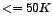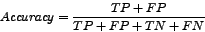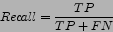DATA MINING
Desktop Survival Guide
by Graham Williams## Measures

True positives (TPs) are those records which are correctly classified by a model as positive instances of the concept being modelled (e.g., the model identifies them as a case of fraud, and they indeed are a case of fraud). False positives (FPs) are classified as positive instances by the model, but in fact are known not to be. Similarly, true negatives (TNs) are those records correctly classified by the model as not being instances of the concept, and false negatives (FNs) are classified as not being instances, but are in fact know to be. These are the basic measures of the performance of a model. These basic measures are often presented in the form of a http://en.wikipedia.org/wiki/Confusion_matrixconfusion matrix, produced using a http://en.wikipedia.org/wiki/contingency_tablecontingency table.

In the following example a simple decision tree model, using rpart, is built using the survey dataset to predict Salary.Group. The model is then applied to the full dataset using predict, to predict the class of each entity (using the type option to specify class rather than the default probabilities for each class). A confusion matrix is then constructed using table to build a contingency table. Note the use of named parameters (Actual and Predicted) to have these names appear in the table.

 > load("survey.RData") > survey.rp <- rpart(Salary.Group ~ ., data=survey) > survey.pred <- predict(survey.rp, data=survey, type="class") > head(survey.pred)  <=50K >50K <=50K <=50K >50K >50K Levels: <=50K >50K > table(Actual=survey\$Salary.Group, Predicted=survey.pred) Predicted Actual <=50K >50K <=50K 23473 1247 >50K 3816 4025 

From this confusion matrix, interpreting the classas the positive class (essentially arbitrarily), we see that there are 23,473 true positives, 4,025 true negatives, 3,816 false positives, and 1,237 false negatives.

Rather than the raw numbers we usually prefer to express these in terms of percentages or rates. The accuracy of a model can, for example, be calculated as the number of entities correctly classified over the total number of entities classified:The recall or true positive rate is the proportion of positive entities which are classified as positive by the model:The recall is a measure of how much of the positive class was actually recovered by the model.